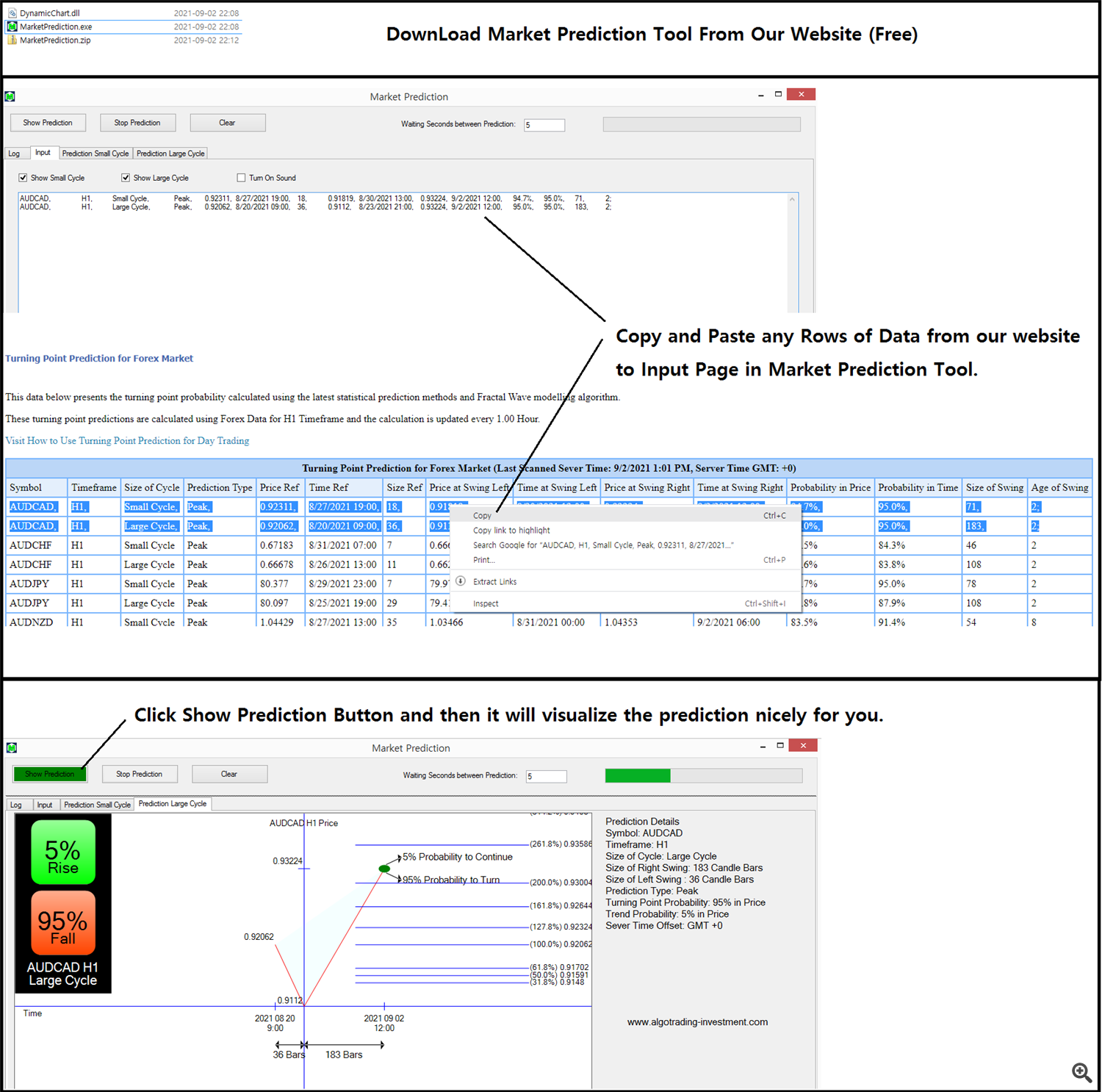# How To Use Forex Prediction With Fibonacci Analysis For Free – Trading Systems – 3 February 2022In this article, we will explain how to use Forex Prediction with Fibonacci Analysis For Free in two steps. In the first step, you can access the Forex Prediction Webpage from the following link. You can book mark this Forex Prediction page below so you can access Forex Prediction any time.

From the above page, you will get access to the turning point prediction for Forex market including EURUSD, GBPUSD, USDJPY, BTCUSD, ETHUSD and so on. In fact, most of statistical predictions are not compatible with the actual trading strategy used by the real world trader. However, the turning point probability (and also the trend probability = 1 – turning point probability) is useful because the predictions were measured from the Fractal Wave. Remember that fractal wave is the direct representation of price action. For example, Support, Resistance, Fibonacci Ratio Analysis, Harmonic Pattern, Elliott Wave Theory, X3 Chart Pattern, Symmetric Triangle Pattern, Rising Wedge Pattern, Falling Wedge pattern, Double Top, Double Bottom, Channel, Renko Chart and so on are such technical analysis derived from Fractal Wave. You can consider the turning point probability as the quantified version of the price patterns. Hence, using the turning point probability with these price pattern is really beneficial in assessing the trend strength and risk for your trading. Especially, we recommend using the turning point probability together with any horizontal support resistance. For example, you can use the hand drawn support resistance, supply demand analysis, round number (=psychological number), pivot analysis, Fibonacci analysis, and so on. I am sure you can think of many horizontal support and resistance of your own.

In the second step, you can visualize the turning point probability and Fibonacci Analysis with this free Market Prediction tool from our website or from google drive. Anyway, you will get the same files.

Download Market Prediction Tool (Free, No Installation, No Signup and Use Straight After Download)

How to use this Market Prediction Tool is simple. Once, you have downloaded the MarketPrediction.zip file, then extract them anywhere in your hard drive. Then run the Market Prediction.exe file. After, you will find a simple user interface. Copy and paste the rows of data from the Market Prediction Webpage from the link. You can copy multiple rows if you wish. Next, click “Show Prediction” button. The Market Prediction software will automatically visualize the turning point probability and trend probability in friendly format.

If you want to use the premium version of the Forex Prediction, then you can use Fractal Pattern Scanner in MetaTrader or Optimum Chart. Below is the landing page for Fractal Pattern Scanner in MetaTrader version, which you can get the real time prediction in MetaTrader.

https://www.mql5.com/en/market/product/49170

https://www.mql5.com/en/market/product/49169